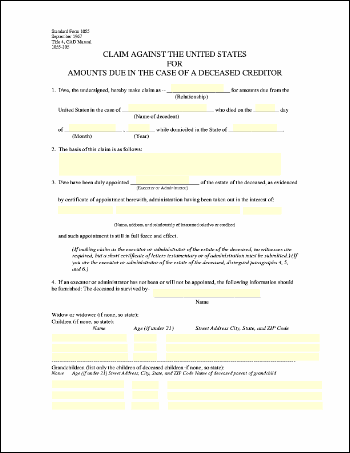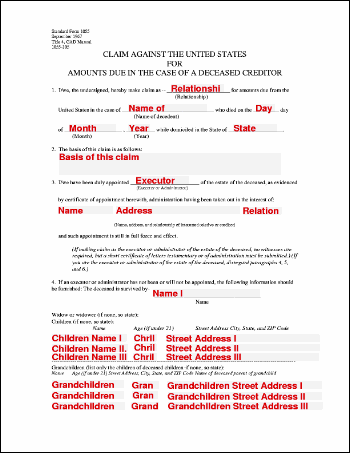eForm Placeholder ExampleThis example shows how to use eForm fields as placeholders for the insertion of text. In this example, we simply replace each of the fields in a form with the name of that field.Src

 First, we create an ABCpdf Doc object and read in our template form. ```Set theDoc = Server.CreateObject("ABCpdf11.Doc") theDoc.Read "c:\mypdfs\form.pdf" theDoc.Font = theDoc.AddFont("Helvetica-Bold") theDoc.FontSize = 16 theDoc.Rect.Pin = 1 ' top left```We iterate through each of the fields. For each field, we focus on the field. We then color the rectangle light gray and draw the name of the field in dark red. ```For Each theField in theDoc.Form.Fields   theField.Focus   theDoc.Color = "240 240 255"   theDoc.FillRect   theDoc.Rect.Height = 16   theDoc.Color = "220 0 0"   theDoc.AddText theField.Name   theDoc.Delete theField.ID Next```Finally, we save. `theDoc.Save "c:\mypdfs\eform.pdf"`Given the following document.form.pdf This is the kind of output you might expect.eform.pdf